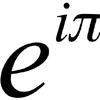#### You may also like### Roots and Coefficients

If xyz = 1 and x+y+z =1/x + 1/y + 1/z show that at least one of these numbers must be 1. Now for the complexity! When are the other numbers real and when are they complex?### 8 Methods for Three by One

This problem in geometry has been solved in no less than EIGHT ways by a pair of students. How would you solve it? How many of their solutions can you follow? How are they the same or different? Which do you like best?### An Introduction to Complex Numbers

A short introduction to complex numbers written primarily for students aged 14 to 19.

# Target Six

##### Age 16 to 18Challenge Level

Show that $x = 1$ is a solution of the equation

$$x^{3/2} - 8x^{-3/2} = 7$$

Find all the solutions of the equation.